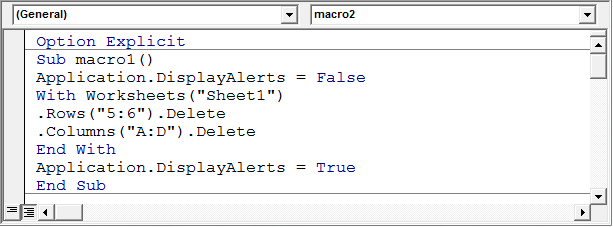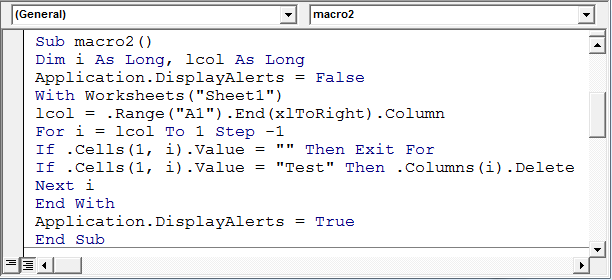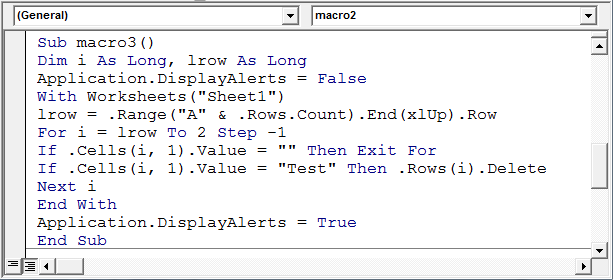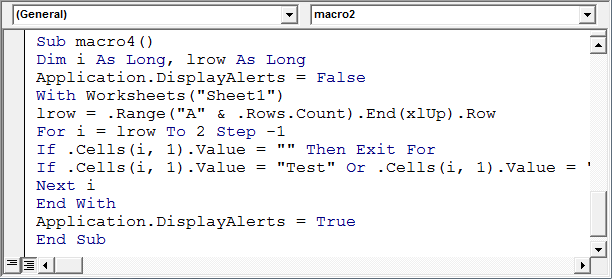# Delete rows and columns using VBA in Microsoft Excel

There could be times when we have to delete blank rows or columns from a file in Excel for loop we will write vba macro / code.  The code that we need to use will vary from situation to situation.  Sometimes we may have to delete one row or column while sometimes we need to delete an entire range of rows or columns or sometimes rows / columns based on one or more conditions.

If you have to delete just one or more specific rows or columns,we use VBA excel for loop and you can just use the following code -

Option Explicit
Sub macro1()
With Worksheets(“Sheet1”)
.Rows(“5:6”).Delete
.Columns(“A:D”).Delete
End With
End Sub

You can see the code which has been copied into the Module1 code window.  To copy the code, press Alt + F11 on your file.  On the left hand side you will see Microsoft Excel Objects.  Right click and select Insert, then click on Module.  The code window will appear on the right.  Copy the code over.  See the below picture -We set display alerts to false and save the file with the required file name.  And then set the display alerts to true just before the End Sub.

With Worksheets(“Sheet1”) is a With loop which ends with End With.  If you use this for loop VBA Excel, all statements inside this loop, which use this object, need not start with “Worksheets(“Sheet1”)”.  Instead, they can directly start with just a dot (.).

.Rows(“5:6”).Delete will delete rows 5 and 6 only.

.Columns(“A:D”).Delete will delete columns A to D.

Only the specified rows / columns will be deleted in this case.

However, sometimes you may have to delete rows / columns based on certain conditions.  For e.g. lets assume that we want to delete all columns in the file which contain “Test” as the header.   In this case, we need a loop which will check from one column to the other and when the criteria is met, it will delete the column.

We can use a code like this.  Note that each person will prepare the code in the manner he/she is comfortable with.  This is just one of the ways this can be coded and is not the only method.

Option Explicit
Sub macro2()
Dim i As Long, lcol As Long
With Worksheets(“Sheet1”)
lcol = .Range(“A1”).End(xlToRight).Column
For i = lcol to 1 Step -1
If .Cells(1,i).Value = “” Then Exit For
If .Cells(1,i).value = “Test” Then .Columns(i).Delete
Next i
End With
End Sub

See the code which has been saved in Module 1 as per the picture belowHere lcol is the last column in the sheet which has data in it.  Incase you happen to test this code on a blank file, lcol will have the value of 16384 or the column number of the last column as per your version of excel.  Then we loop from the last column to the first column, moving one step backwards each time.  We are moving backwards because the delete code works fine only when you start deleting from the last row or last column.  If you start from the 1st row / column the code tends to error out.

The 1st if condition is input there incase you run the macro on a blank file.  In Excel macro will loop from the last column which is 16384 in this case till column 1 and this could take a fairly long time.  So incase the code finds a blank column, it will terminate the loop immediately through the exit For statement.  The 2ndif condition checks if the value is “Test” and deletes the column if “Test” is found.

In case you want to delete the rows instead of columns, the code will change to –

Option Explicit
Sub macro3()
Dim i As Long, lrow As Long
With Worksheets(“Sheet1”)
lrow = .range(“A” & .Rows.Count).End(xlUp).Row
For i = lrow to 2 Step -1
If .Cells(i,1).Value = “” Then Exit For
If .Cells(i,1).Value = “Test” Then .Rows(i).Delete
Next i
End With
End Sub

See the code below -You would have observed that the .Cells(1,i).value changes to .Cells(i,1).value in the above code.  This happens because in the first code, we were deleting columns so the variable “i” had to loop through the columns, while in the 2nd code, it needs to loop through the rows.  Here we will loop from the last row to the 2nd row, as the 1st row contains headers which need to be retained.

As per the code requirement, you can even merge both the delete rows and delete columns code into 1 code.  You can even put in multiple conditions based on which the row / column should be deleted.  Consider, we want to delete all rows where the 1st column contains text such as Test or Dummy, then the code will be like this –

Option Explicit
Sub macro4()
Dim i As Long, lrow As Long
With Worksheets(“Sheet1”)
lrow = .range(“A” & .Rows.Count).End(xlUp).Row
For i = lrow to 2 Step -1
If .Cells(i,1).Value = “” Then Exit For
If .Cells(i,1).Value = “Test”  Or .Cells(i,1).Value = “Dummy” Then .Rows(i).Delete
Next i
End With
End SubSimilarly, you can add on to this code or the code you have based on the requirement. You can add in multiple conditions for rows and even for columns.1."Hi,